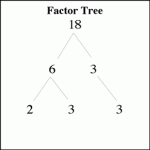GCD Calculator – About GDC

Greatest Common Divisor is the largest positive integer number that divides the numbers with no remainder. GCDCalculator will help you to find this number. The GCD is helpful for reducing fractions to be in lowest terms.

GCD Calculator

How to Find the Greatest Common Divisor without GCD Calculator?

If you can’t use the GCD Calculator, like in a activity in a classroom, you can do it manually.

GDC CalculatorSteps:

1. Cancel any negative signs.

2. Discover the bigger of the two numbers. That will be the D (Dividend), and the smaller the Di(Divisor).

3. Write out this formula: (D) = (DI) * (Q) + (R)

4. Put the bigger number in the spot for dividend, and the smaller number as the divisor.

5. Choose how many times the smaller number will divide into the larger number, and drop it into the formula as the Q.

6. Calculate the R (Residue), and substitute it into the appropriate place in the formula.

7. Write out the formula again, but this time A) use the old divisor as the new dividend and B) use the remainder as the new divisor.

8. Do again the previous step until the residue is zero.

9. The final divisor is the GCD.

Without the GCD Calculator. You can also use the LCM Calculator !